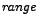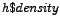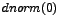DATA MINING
Desktop Survival Guide
by Graham Williams## Basic Histogram with Density Curve

R allows plots to be built up--this example shows a density histogram of a set of random numbers extracted from a normal distribution with the density curve of the same normal distribution also displayed. In the R code we build the histogram at first without plotting it, so as to determine the y limits (selects the minimum and maximum values, whileis the list of density values being plotted andis the maximum possible value of the density), since otherwise the curve might push up into the title!

[width=0.8]rplot-hist-density

 ```ds <- rnorm(200) pdf("graphics/rplot-hist-density.pdf") par(xpd=T) h <- hist(ds, plot=F) ylim <- range(0, h\$density, dnorm(0)) hist(ds, xlab="normal", ylim=ylim, freq=F, main="Histogram of Normal Distribution with Density") curve(dnorm, col=2, add=T) dev.off() ```

http://rattle.togaware.com/code/rplot-hist-density.R